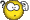# LFSR32Rand: A new Random Number Generator for JackClassicListThreaded7 messagesOpen this post in threaded view
|

## LFSR32Rand: A new Random Number Generator for Jack

This post was updated on .
[2016-16-13 Updated expected value table in README.txt in zip file.]

### LFSR32Rand: A new Random Number Generator for Jack

sorbus contributed a nice Random Number Generator in this post. It's fast and works well for the limited use of random numbers required for most games.

If you are writing a program that needs a large number of random numbers, more than 32K of them, you will run into trouble due to the cycle length limitation in 16-bit Linear Congruential Generator based random number generators.

Here is a new Random number generator that is somewhat slower than LCGRandom, but has a cycle length of 232-1 (4 billion), and has better randomness than LCG-based random number generators.

LFSR32Rand source code and test application:
LFSR32Rand-1-1.zip

I'll write a theory of operations for LFSR32Rand in a followup post.

--Mark

```Contents:
--------

LFSR32Rand.jack     LFSR-based random number generator.

Main.jack           Test program for LFSR32Rand -- presents side by side test
patterns drawn with LCGRand and LFSR32Rand.
LCGRand.jack        "Objectized" version of LCGRandom.jack.  (Used by Main, not
required by LFSR32Rand.)

LFSR32Rand uses a Linear Feedback Shift Register to implement a random number
generator with a cycle length of 2^32-1 (4.29e+9).

LFSR32Rand solves several problems that can occur when using LCG-based random
number generators like LCGRandom.

1) Because Hack only supports 16-bit signed multiplication, the LCG has a
cycle length of at most 32767 values before it repeats.
2) LCGs have poor randomness in the lower bits of the generated numbers.
3) LCGs can have noticeable patterns (autocorrelation) between returned values.
4) The LCG's unscaled values are unique; there are no duplicate values in the
series returned by rand().  True random values have randomly occurring

Test program notes:
------------------

The "mormal-ish" distribution is the sum of three uniformly distributed random
integers in the range 0-85 (3d86).

The number displayed at the end of the test is the number of black pixels at
the end of the test.

Interesting tests to look at:

Uniform distribution, 16K, set pixels -- Beginning to see LCG patterning.
Uniform distribution, 32K, set pixels -- Strong patterning, overly dense.
Uniform distribution, 64K, XOR pixels -- Pixels turned off during 2nd LCG cycle.
Normal distribution, 16K, set pixels -- Diagonal patterning, low center density.
Random walk, 32K, set pixels -- LCG draws an interesting creature.
Random walk, 64K, XOR pixels -- The creature gets drawn and erased.

(See Nand2Tetris forum
for information about why the LCG generates the creature.)

Expected Value Table:
--------------------

n(K)          set pixel               XOR pixel
uniform      3d86       uniform      3d86
1      1016.05     1002.95     1008.18      982.64
2      2016.35     1965.24     1985.34     1888.10
4      3970.65     3776.14     3850.40     3497.60
8      7700.74     6995.09     7248.35     6078.50
16     14496.61    12156.86    12893.35     9585.08
32     25786.56    19170.01    20713.51    13458.44
64     41426.84    26916.78    28333.47    17109.61
128     56666.80    34219.17    32167.87    20318.87
256     64335.70    40637.71    32757.01    23034.44
512     65514.02    46068.88    32768.00    25269.91
```
Open this post in threaded view
|

## LFSR32Rand: Theory of operation

### LFSR32Rand: Theory of operation

#### Preliminaries — Shift Registers

A shift register is a register with the added capability of modifying its stored data by shifting it one bit to the left or right. Shift registers are often used to convert parallel data to serial bit streams and vice versa. Shift registers can have serial-input, serial-output, parallel-input and parallel-output in various combinations depending on their task.

The most generic shift register has both types of inputs and outputs. Here is an example shift register schematic and HDL file: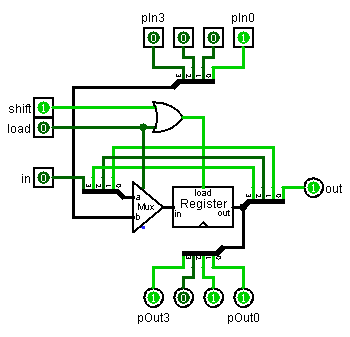4-bit [right] Shift Register

```/**
*  16-bit Shift Register (left-shifting).
*/

CHIP ShiftReg16 {
IN  in,         // Serial input
pIn,    // Parallel input
shift,      // True to shift when clocked
OUT out,        // Serial output
pOut;   // Parallel output
PARTS:
a=in, a[1..15]=regOut,     // regIn = (regOut<<1) | in
b=pIn);                       // regIn = pIn
}
```
The LFSR32Rand-theory.zip file (bottom of post) contains this HDL and a test file to exercise it.

#### Linear Feedback Shift Register

A linear feedback shift register connects some of its parallel outputs back to its serial input through a network of XOR gates. Here is a schematic of a 4-bit LFSR (uses the 4-bit shift register shown above):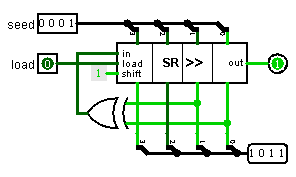4-bit Linear Feedback Shift Register

This LFSR cycles through this sequence:

```0001   1000   0100   0010   1001   1100   0110   1011
0101   1010   1101   1110   1111   0111   0011  (0001)
```
Notice that the value 0000 is missing from the cycle. If the LFSR contains all zeros, it will never change.

The LFSR32Rand-theory.zip file (bottom of post) contains HDL and test files for this 4-bit LFSR and a 32-bit LFSR that is the LFSR used by LFSR32Rand.

#### LFSR32Rand.jack

The fundamental data structure in LFSR32Rand is a 32-bit shift register comprised of the two 16-bit fields msw and lsw

Left shifting the double-word register requires three steps:
• msw must be shifted by doubling.
• msw bit 0 must be set to lsw bit 15.
• lsw must be shifted by doubling.

The Jack code to do this uses the < comparison to test if bit 15 is set, and takes advantage of the comparison result being -1 (true) or 0 (false).

```let msw = (msw+msw) - (lsw < 0);    // '<' returns -1 if true
let lsw = lsw+lsw;
```

The fundamental function in LFSR32Rand is randBit() which generates the next random bit that will be used to compose the random numbers.

It computes the next input bit to the LFSR and then shifts the LFSR to the left and inserts the new bit.

Jack (and Hack) is not good at XOR, so randBit() uses the fact that XORing multiple bits together is the same as adding them modulo 2. The code is rather sneaky in that it adds true and false values as surrogates for the individual bits that need to be XORed.

```let bit = ( (msw < 0)               // true if 0x80000000 set
+ ((msw & 64) = 64)       // true if 0x00400000 set
+ ((lsw & 4096) = 4096)   // true if 0x00001000 set
+ ((lsw & 32) = 32)       // true if 0x00000020 set
) & 1;
```

#### Example Files

Note that the schematics in this post show right-shifting circuits, but the example HDL files are left-shifting

LFSR32Rand-theory-1-0.zip contains:

 ShiftReg4.cmp 4-bit shift register ShiftReg4.hdl ShiftReg4.tst ShiftReg16.cmp 16-bit shift register ShiftReg16.hdl ShiftReg16.tst LFSR4.cmp 4-bit linear feedback shift register LFSR4.hdl LFSR4.tst LFSR32.cmp 32-bit linear feedback shift register LFSR32.hdl LFSR32.tst
Open this post in threaded view
|

## Re: LFSR32Rand: Theory of operation

 This is awesome!
Open this post in threaded view
|

## Re: LFSR32Rand: A new Random Number Generator for Jack

 I agree. I got this frog thing: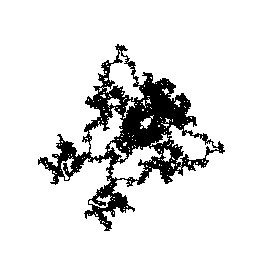I have to say, this is a good one. 😀
Open this post in threaded view
|

## Re: LFSR32Rand: A new Random Number Generator for Jack

 In reply to this post by cadet1620 And this is also useful for my tetris game. 😎
 Now the LFSR32Rand random number generator is making an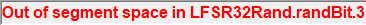Error. What's wrong?